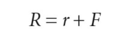# Fisher effect

## Fisher effect

A theory that nominal interest rates in two or more countries should be equal to the required real rate of return to investors plus compensation for the expected amount of inflation in each country.

## Fisher Effect

A theory stating that real interest rates are independent of monetary considerations. According to the Fisher effect, a currency's real interest rate is equal to its nominal interest rate less the inflation rate. Thus, if the inflation rate rises, the real interest rate eventually rises as well; likewise, if inflation falls, the real interest rate will fall. See also: International Fisher Effect.

## Fisher effect

The direct relationship between inflation and interest rates. Increasing inflationary expectations result in increasing interest rates.

## Fisher effect

an expression that formally allows for the effects of INFLATION upon the INTEREST RATE of a LOAN or BOND. The Fisher equation, devised by Irving Fisher (1867–1947), expresses the nominal interest rate on a loan as the sum of the REAL INTEREST RATE and the rate of inflation expected over the duration of the loan:where R = nominal interest rate, r = real interest rate and F = rate of annual inflation. For example, if inflation is 6% in one year and the real interest rate required by lenders is 4%, then the nominal interest rate will be 10%. The inflation premium of 6% incorporated in the nominal interest rate serves to compensate lenders for the reduced value of the currency loaned when it is returned by borrowers.

The Fisher effect suggests a direct relationship between inflation and nominal interest rates, changes in annual inflation rates leading to matching changes in nominal interest rates. See INTERNATIONAL FISHER EFFECT.

Collins Dictionary of Economics, 4th ed. © C. Pass, B. Lowes, L. Davies 2005
References in periodicals archive ?
The reason being the pricing of the loan, or interest rate, is subject to the Fisher effect (Irving Fisher, 1930), arising directly from the so-called Fisher equation, to wit, interestnominal= interestreal + inflation expectations, which is the template for all equations in finance.
Typically, this is explained as arising from the Fisher effect, named after Irving Fisher, whereby higher inflation increases nominal interest rates through an inflation premium effect.
When the Fisher effect is applied to the stock returns/inflation relationship, it is suggested that stocks would move one-to-one with inflation, thus making stocks a hedge for inflation.
But every macroeconomist knows about the Fisher effect, whereby a reduction in the nominal interest rate reduces inflation--in the long run.
This Fisher effect is well known and is not likely to be disputed in macroeconomic circles.
interest rates affect the multinational corporation through the Fisher Effect, the International Fisher Effect, and interest rate parity relationships.
Evidence in some of the empirical studies, mentioned earlier, rejected the strict Fisher effect, but found less than complete adjustment of nominal interest rate to changes in inflation rates.
Empirical findings obtained from this approach are abundant but inconclusive thus far; see Cooray (2003) and Johnson (2006) who provide excellent overviews of the theoretical and empirical issues on the Fisher effect.
If the level of inflation increases, interest rates will increase as well (the so-called Fisher effect), lowering the value of fixed-income assets.
First, in terms of anticipated component of inflation, we find that the Fisher effect appears to hold at short horizons, where expected inflation has insignificant short-run impacts on real stock returns but not at longer horizons and where anticipated inflation is found to have negative long-run impacts on real stock returns.
Coach-loads of people are travelling from Wales to the West End once more thanks to the 'Connie Fisher effect' boosting the popularity of musicals.

Site: Follow: Share:
Open / Close